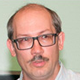## Joint Chinese-Russian Mathematical Online Colloquium

##### 11:00 (GMT+3)Ying Zhang Soochow University Bio: Ying Zhang obtained his Ph.D. from the National University of Singapore. He is currently a professor of mathematics at Soochow University. His research interests include hyperbolic geometry and low-dimensional topology. He has contributed to the geometry of hyperbolic cone-surfaces, generalizations of McShane identity, dynamics and topology of the character varieties of surfaces, trigonometry of 4-dimensional hyperbolic space etc.

### Trace polynomials for closed curves on the enlarged modular torus: Monotonicity, positivity, and log-concavity.

The modular torus is a once-cusped hyperbolic torus with the maximal order of symmetry. The traces of the simple closed geodesics on the modular torus give the geometric version of the classical Markoff numbers. We study trace polynomials for the closed curves on the enlarged modular torus, with the single variable the enlargement factor. We obtain partial ordering of the polynomials for certain simple closed curves. We obtain positivity of the polynomials for all closed curves. We have observed log-concavity for these polynomials and confirmed log-concavity of the polynomials for the simple closed curves. We also mention the problem to enlarge an arbitrary complete one-holed hyperbolic torus. This is joint work with Xiangfei Li.

##### 12:00 (GMT+3)Ivan A. Dynnikov Steklov Mathematical Institute of RAS Bio: Ivan A. Dynnikov is a Professor at the Department of Higher Geometry and Topology, Lomonosov Moscow State University and also a Leading Scientific Researcher at Steklov Mathematical Institute of RAS. He obtained his PhD from Lomonosov Moscow State University in 1996 and became Doctor of Sciences in 2007. Dynnikov's research interests include low-dimensional topology and its applications in mathematical physics, knot theory, discrete Schrödinger operators, and the theory of dynamical systems. He was an invited speaker at the 2nd European Congress of Mathematicians (Budapest, 1996). In 2000, I.A. Dynnikov was awarded the prize of the Moscow Mathematical Society for young scientists. I.A. Dynnikov is the Scientific Secretary of the Moscow Mathematical Society and the author of more than 35 scientific publications.

### Diagrammatic approach to the classification of knots and contact topology.

About two decades ago I discovered that the problem of recognizing the unknot could be solved using a monotonic simplification procedure for certain type knot diagrams, which I called rectangular. They are also now known as grid diagrams. For arbitrary knots, the procedure does not work as well as it does for the unknot, since there may be many non-equivalent rectangular diagrams representing the same knot type that are not subject to any simplification by elementary moves. However, for many knot types, the set of non-simplifiable diagrams is finite, and it does not seem impossible that this is true for all knots. The classification of non-simplifiable rectangular diagrams turns out to be deeply related to the classification of Legendrian knots. This relation has been recently studied in our joint works with Maxim Prasolov and Vladimir Shastin. As a by-product of this study, we obtained an algorithm for comparing Legendrian knots, which has not been known before. In my talk, I will overview the most recent results on the subject.

The present work is supported by the Russian Science Foundation under grant~22-11-00299.

The meeting will be held in the form of a webinar on the Zoom platform.

Pre-registration for the event is not required.